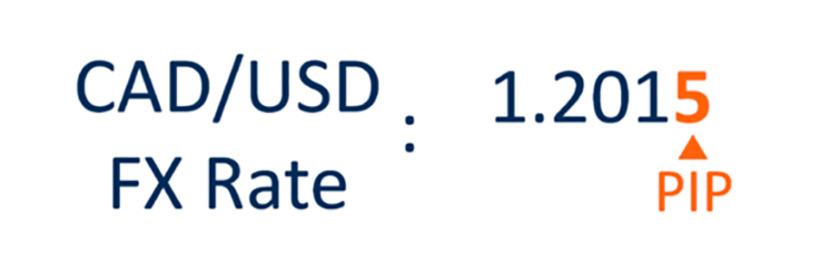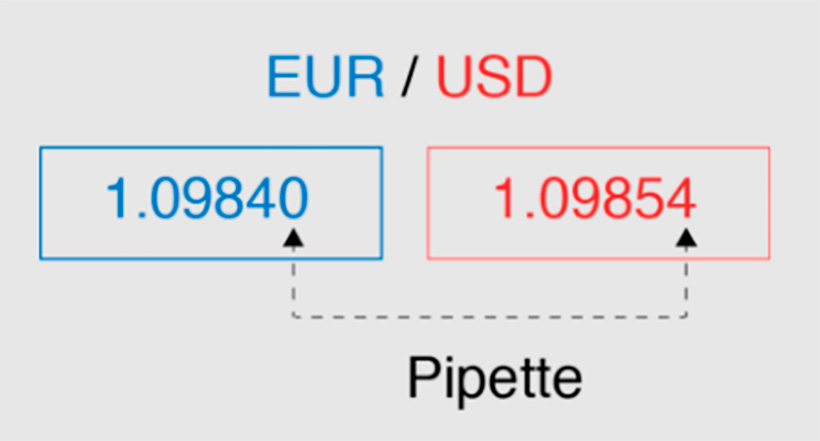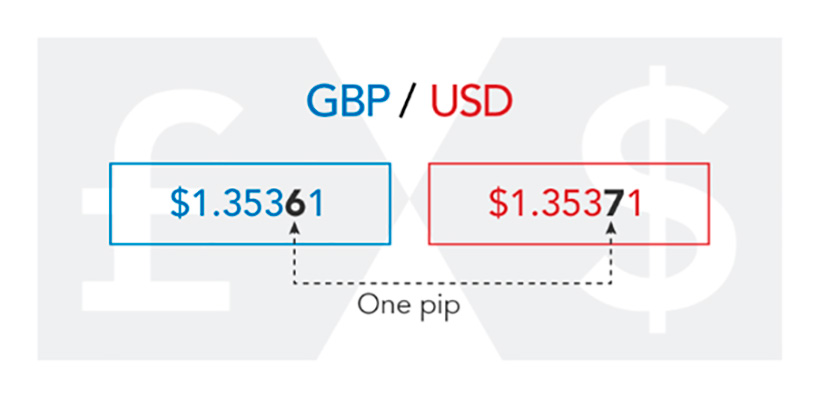Pip Forex is an abbreviation that stands for "percentage price" or "percentage in point." In the Forex market, this term is used quite often, meaning the smallest price change. Simply put, a pip is synonymous with the term "basis point" (bps). That is, when we define what does pip mean in Forex, we mean exactly such definitions.

But let's understand not only what are Forex pips but also how to count pips in Forex, and why it is important to understand the pips definition Forex before you start working in the market.

## What Is a Pip?Let's start with what is a pip in Forex. Pips help to understand the change in the value of one currency relative to another, that is, it is a specific unit of measurement convenient for traders and investors. Why is it useful to know what pips are in Forex trading? This term allows specialists to quickly navigate changes in the market without lengthy explanations. However, pips are not just a unit of measurement.

Usually, understanding pips in Forex means the last decimal place in the currency price quote. For example, when the CAD/USD price was 1.2014 and became 1.2015, this difference of \$0.0001 is a change of one point. Here is the answer to the question of how much is a pip in Forex — 0.0001 dollars. It also happens that the pip definition Forex means the second sign after the comma. For example, the Japanese yen has only two decimal places, so as an exception, in this case, a change of 0.01 will be considered pips.

Some Forex brokers go beyond the traditional 4 or 2 decimal places. And what are pips in Forex in this case? Here, we are talking about 3 or 5 decimal places. Knowing how much is 1 pip in Forex and how to use it gives you a competitive advantage because you see the situation more broadly than your competitors and, therefore, achieve more.A pip seems to be a very small particle, with a minimal change in value. But on Forex, there are even smaller values. It is about the so-called pipette. You might also hear the names dot or fractional pip. A pipette is one-tenth of a pip. To newbies, this seems like an insignificant little thing and can be ignored because, when we are talking about an increase or decrease in price by 0.00001 USD, that is, by 1 dropper, this is not a significant change at all. However, investors pay attention to it when it comes to trends.

Then, when the price has not yet moved by 1 basis point, the pipette allows you to understand whether there will be such changes in the future and how exactly the market situation will turn out. You have to follow such small things so as not to lose a lot of your assets and open positions. It also allows the trader to spot a profit opportunity before their colleagues spot a certain trend.

## Pip: How does it work?

Forex currency markets are highly liquid. A large volume of transactions is carried out here every day, so it is worth keeping a close eye on what is happening. By receiving the most accurate data, it is easier for the investor to predict the further movement of the market and plan trading tactics. However, pips are not used all the time. It is advisable to do this in case of hyperinflation of currencies: in such moments, exchange rates become uninformative, and it is difficult to evaluate them correctly. With intensive FX movement, there is little benefit from following pips. Optimally, it is when a trader understands pip meaning Forex and turns it into a reliable working tool, given the appropriate context.

In the case of hyperinflation, pip in Forex becomes not an objective indicator for evaluating exchange rate fluctuations: prices rise excessively, are not controlled, and the movement becomes intense.

### What does pip stand for in Forex?

You can use this indicator to estimate the bid-ask spread, i.e., the price difference between the buyer and the seller. However, the main purpose of pips is to evaluate trading results and predict future actions.

### Risk management on Forex: What is a pip role?

You can also use pips calculations to reduce potential risk. Specifically, you can do the following:

• Determine the risk of the transaction as a percentage of the balance.
• Place a stop loss, expressing it in points.
• Determine the ideal position size.

## How to count pips?A point is 0.0001. But its exact value each time depends on a specific currency pair, the exchange rate set at a certain moment in time, and the trading value. Therefore, if you are trying to understand how to count pips Forex, you should take into account the three criteria mentioned above.

### How do such calculations look in practice?

Let's figure out how to count pips in Forex using real examples.

In the case of an equal EUR/USD pair, a simplified calculation formula applies. To find out how much is a pip in Forex for this pair, you need to multiply the trade value or lot size by 0.0001. For example, if the value of the deal is \$10,000, we get:

10,000 × 0.0001 = \$1.

So, the cost of one item in this case is \$1. What does such information give the investor? If they bought 10,000 EUR and then sold it when the price went up by 1 point, their actual profit is 10 USD.

When USD is the first currency in the pair, that is, the base currency, the current exchange rate for this pair should also be included in the value of the item. In this case, the formula looks like this:

0.0001 (or another item size, depending on the situation) / per currency pair exchange rate * transaction value

Since this formula looks much more complicated, let's try to make calculations on the example of the USD/CAD currency pair.

Data for calculations:

• The point size is 0.0001
• USD/CAD exchange rate — 1.2827
• The lot’s size is standard, 100,000 USD

Calculations:

0,0001/1,2827 × 100 000=7,79

That is, under the given conditions, the cost of one item is \$7.79. Thus, if a trader buys 100,000 USD per Canadian dollar at the rate of 1.2827 and sells at the rate of 1.2828, the net profit on this transaction will be \$7.79. Please note that the cost of an item is not a constant value. It changes depending on how the price fluctuates. In the considered examples, we do not see very large amounts, but traders conduct many transactions and deal with much larger trading volumes, so the difference in each point is the real profit or loss of the trader.

### Japanese yen: How to calculate the cost of an item?

How to calculate pips on Forex in the case of such a currency as the Japanese yen? JPY is an exception to the 4 decimal places rule. This can cause difficulties in calculations.

In this case, the calculation of Forex pips is explained as follows:

Cost of 1 pips = 1/100/ current exchange rate * lot size

Example and data for Forex trading pip calculations in the EUR/JPY pair:

• Currency quotation — 132.62;
• The lot's size is 100,000 euros.

Calculation:

1:100:132,62 × 100 000=7,54

So, pips meaning Forex is expressed in specific numbers that traders pay attention to when opening and closing positions, as well as before opening trades. Profitability or loss of a few pips is expressed in very real amounts in the investor's accounts. That is why it is so important to know how to calculate pips in Forex and bring this skill to automaticity (or use special online calculators).

## The Bottom Line

Now, you know exactly what is pips in Forex, and this allows you to objectively evaluate the results of each transaction and make correct predictions. Also, the definition of what's a pip in Forex helps to effectively manage risks and adjust your actions according to the market situation.